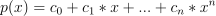/NumPy 1.17

# numpy.polynomial.polynomial.polyval

`numpy.polynomial.polynomial.polyval(x, c, tensor=True)` [source]

Evaluate a polynomial at points x.

If `c` is of length `n + 1`, this function returns the valueThe parameter `x` is converted to an array only if it is a tuple or a list, otherwise it is treated as a scalar. In either case, either `x` or its elements must support multiplication and addition both with themselves and with the elements of `c`.

If `c` is a 1-D array, then `p(x)` will have the same shape as `x`. If `c` is multidimensional, then the shape of the result depends on the value of `tensor`. If `tensor` is true the shape will be c.shape[1:] + x.shape. If `tensor` is false the shape will be c.shape[1:]. Note that scalars have shape (,).

Trailing zeros in the coefficients will be used in the evaluation, so they should be avoided if efficiency is a concern.

Parameters: `x : array_like, compatible object` If `x` is a list or tuple, it is converted to an ndarray, otherwise it is left unchanged and treated as a scalar. In either case, `x` or its elements must support addition and multiplication with with themselves and with the elements of `c`. `c : array_like` Array of coefficients ordered so that the coefficients for terms of degree n are contained in c[n]. If `c` is multidimensional the remaining indices enumerate multiple polynomials. In the two dimensional case the coefficients may be thought of as stored in the columns of `c`. `tensor : boolean, optional` If True, the shape of the coefficient array is extended with ones on the right, one for each dimension of `x`. Scalars have dimension 0 for this action. The result is that every column of coefficients in `c` is evaluated for every element of `x`. If False, `x` is broadcast over the columns of `c` for the evaluation. This keyword is useful when `c` is multidimensional. The default value is True. New in version 1.7.0. `values : ndarray, compatible object` The shape of the returned array is described above.

#### Notes

The evaluation uses Horner’s method.

#### Examples

```>>> from numpy.polynomial.polynomial import polyval
>>> polyval(1, [1,2,3])
6.0
>>> a = np.arange(4).reshape(2,2)
>>> a
array([[0, 1],
[2, 3]])
>>> polyval(a, [1,2,3])
array([[ 1.,   6.],
[17.,  34.]])
>>> coef = np.arange(4).reshape(2,2) # multidimensional coefficients
>>> coef
array([[0, 1],
[2, 3]])
>>> polyval([1,2], coef, tensor=True)
array([[2.,  4.],
[4.,  7.]])
>>> polyval([1,2], coef, tensor=False)
array([2.,  7.])
```

© 2005–2019 NumPy Developers
Licensed under the 3-clause BSD License.
https://docs.scipy.org/doc/numpy-1.17.0/reference/generated/numpy.polynomial.polynomial.polyval.html